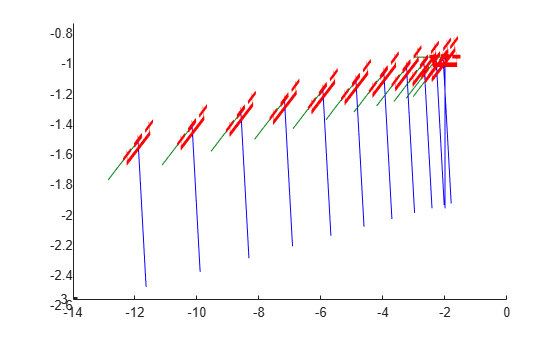multirotor

Guidance model for multirotor UAVs

Description

A multirotor object represents a reduced-order guidance model for an unmanned aerial vehicle (UAV). The model approximates the behavior of a closed-loop system consisting of an autopilot controller and a multirotor kinematic model for 3-D motion.

For fixed-wing UAVs, see fixedwing.

Creation

model = multirotor creates a multirotor motion model with double precision values for inputs, outputs, and configuration parameters of the guidance model.

model = multirotor(DataType) specifies the data type precision (DataType property) for the inputs, outputs, and configurations parameters of the guidance model.

Properties

expand all

Name of the UAV, used to differentiate it from other models in the workspace, specified as a string scalar.

Example: "myUAV1"

Data Types: string

UAV controller configuration, specified as a structure of parameters. Specify these parameters to tune the internal control behaviour of the UAV. Specify the proportional (P) and derivative (D) gains for the dynamic model and other UAV parameters. For multirotor UAVs, the structure contains these fields with defaults listed:

• 'PDRoll'- [3402.97 116.67]

• 'PDPitch' - [3402.97 116.67]

• 'PYawRate' - 1950

• 'PThrust' - 3900

• 'Mass' - 0.1 (measured in kg)

Example: struct('PDRoll',[3402.97,116.67],'PDPitch',[3402.97, 116.67],'PYawRate',1950,'PThrust',3900,'Mass',0.1)

Data Types: struct

UAV guidance model type, specified as 'MultirotorGuidance'.

Input and output numeric data types, specified as either 'double' or 'single'. Choose the data type based on possible software or hardware limitations. Specify DataType when first creating the object.

Object Functions

 control Control commands for UAV derivative Time derivative of UAV states environment Environmental inputs for UAV state UAV state vector

Examples

collapse all

This example shows how to use the multirotor guidance model to simulate the change in state of a UAV due to a command input.

Create the multirotor guidance model.

model = multirotor;

Create a state structure. Specify the location in world coordinates.

s = state(model);
s(1:3) = [3;2;1];

Specify a control command, u, that specified the roll and thrust of the multirotor.

u = control(model);
u.Roll = pi/12;
u.Thrust = 1;

Create a default environment without wind.

e = environment(model);

Compute the time derivative of the state given the current state, control command, and environment.

sdot = derivative(model,s,u,e);

Simulate the UAV state using ode45 integration. The y field outputs the multirotor UAV states as a 13-by-n matrix.

simOut = ode45(@(~,x)derivative(model,x,u,e), [0 3], s);
size(simOut.y)
ans = 1×2

13        3536

Plot the change in roll angle based on the simulation output. The roll angle (the X Euler angle) is the 9th row of the simOut.y output.

plot(simOut.y(9,:))Plot the change in the Y and Z positions. With the specified thrust and roll angle, the multirotor should fly over and lose some altitude. A positive value for Z is expected as positive Z is down.

figure
plot(simOut.y(2,:));
hold on
plot(simOut.y(3,:));
legend('Y-position','Z-position')
hold offYou can also plot the multirotor trajectory using plotTransforms. Create the translation and rotation vectors from the simulated state. Downsample (every 300th element) and transpose the simOut elements, and convert the Euler angles to quaternions. Specify the mesh as the multirotor.stl file and the positive Z-direction as "down". The displayed view shows the UAV translating in the Y-direction and losing altitude.

translations = simOut.y(1:3,1:300:end)'; % xyz position
rotations = eul2quat(simOut.y(7:9,1:300:end)'); % ZYX Euler
plotTransforms(translations,rotations,...
'MeshFilePath','multirotor.stl','InertialZDirection',"down")
view([90.00 -0.60])